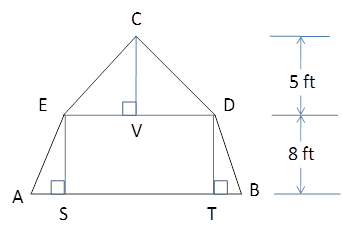Chapter 4.4, Problem 37E### Elementary Geometry for College St...

6th Edition
Daniel C. Alexander + 1 other
ISBN: 9781285195698

#### Solutions

Chapter
Section### Elementary Geometry for College St...

6th Edition
Daniel C. Alexander + 1 other
ISBN: 9781285195698
Textbook Problem
3 views

# In a gambrel style roof, the gable end of a barn has the shape of an isosceles trapezoid surmounted by an isosceles triangle. If AE=30 ft and BD=24 ft, find: (a) AS (b) VD (c) CD (d) DETo determine

(a)

To Find:

AS

Explanation

Consider the following diagram.

From the given diagram, we know that BSAEandDTAE.

Therefore, BD=ST.

It is given that BD=24ft.

Then, ST=24ft.

Since, ABDE is an isosceles trapezoid ΔABSΔEDT.

Then, AS=TE

To determine

(b)

To Find:

VD

To determine

(c)

To Find:

CD

To determine

(d)

To Find:

DE

### Still sussing out bartleby?

Check out a sample textbook solution.

See a sample solution

#### The Solution to Your Study Problems

Bartleby provides explanations to thousands of textbook problems written by our experts, many with advanced degrees!

Get Started